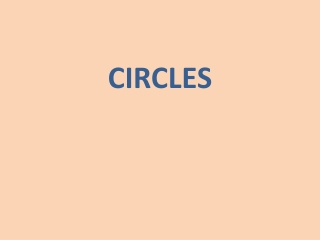DownloadDownload PresentationCIRCLES

# CIRCLES

Download Presentation## CIRCLES

- - - - - - - - - - - - - - - - - - - - - - - - - - - E N D - - - - - - - - - - - - - - - - - - - - - - - - - - -
##### Presentation Transcript

1. CIRCLES

2. SPECIFIC OBJECTIVES: At the end of the lesson, the student is expected to be able to: • draw a circle given different points. • determine center and radius of the circle given an equation. • determine general and standard form of equation of the circle given some geometric conditions. • convert general form to standard form of equation of the circle and vice versa.

3. CIRCLE A circle is a locus of points that moves in a plane at a constant distance from a fixed point. The fixed point is called the center and the distance from the center to any point on the circle is called the radius. Parts of a Circle Center - It is in the centre of the circle and the distance from this point to any other point on the circumference is the same. Radius - The distance from the centre to any point on the circle is called the radius. A diameter is twice the distance of a radius. Circumference - The distance around a circle is its circumference. It is also the perimeter of the circle

4. Chord - A chord is a straight line joining two points on the circumference. The longest chord in a called a diameter. The diameter passed through the centre. Segment - A segment of a circle is the region enclosed by a chord and an arc of the circle. Secant - A secant is a straight line cutting at two distinct points. Tangent - If a straight line and a circle have only one point of contact, then that line is called a tangent. A tangent is always perpendicular to the radius drawn to the point of contact.

5. Equation of a Circle

6. Let: C (h, k) - coordinates of the center of the circle r - radius of the circle P (x, y) - coordinates of any point along the circle From the figure, Distance CP = radius ( r ) Recall the distance formula: Squaring both sides of the equation, r2 = (x – h)2 + (y – k)2 The equation is also called the center-radius form or the Standard Form. (x – h)2 + (y – k)2 = r2

7. If the center of the circle is at the origin (0, 0) h = 0 k = 0 C (h, k) C (0, 0) From (x – h)2 + (y – k)2 = r2 (x – 0)2 + (y – 0)2 = r2 x2 + y2 = r2 Center at the origin

8. From (x – h)2 + (y – k)2 = r2 Standard Form Center at (h, k) (x2 – 2hx + h2) + (y2 – 2kx + k2) = r2 x2 + y2 – 2hx – 2ky + h2 + k2 + r2= 0 Let: 2h = D 2k = E CONSTANTS h2 + k2 + r2 = F Therefore, x2 + y2 + Dx + Ey + F = 0General Form

9. Examples: If the center of the circle is at C(3, 2) and the radius is 4 units, find the equation of the circleand sketch the graph. Reduce to standard form and draw the circle whose equation is 4x2 + 4y2 – 8x – 8y – 16 = 0. Determine the center and radius of the circle (x + 3)2 + (y – 2)2 = 16. Sketch the graph. Reduce x2+ y2– 18x + 10y + 25 = 0 to the center-radius form of the circle. Give the standard form for the equation of a circle with center (2, –4) and radius 5. Find the equation of the circle having (–1, –3) and (7, –1) as ends of a diameter.